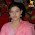Given an integer n, write a function that returns count of trailing zeroes in n!.
```Examples:
Input: n = 5
Output: 1
Factorial of 5 is 20 which has one trailing 0.

Input: n = 20
Output: 4
Factorial of 20 is 2432902008176640000 which has
4 trailing zeroes.

Input: n = 100
Output: 24
```

### Comments

1.Nice Website...
Hey JOIN now fblikesbot.com and Increase Facebook Likes your profile and websites.
Increase Facebook Likes and check your website worth worth my websites
its may be very beneficial for you also really

2.#include
#include
int factorial(int n);
int count(int c);
main()
{
int n,counter=0;
long fact;
printf("Enter Any number :\n");
scanf("%d",&n);
if(n<1){
printf("Please enter greater than 1");
}
fact = factorial(n);
printf("Factorial of %d is %ld\n",n,fact);
counter = count(fact);
printf("Which has %d Zeroes",counter);
getch();
}

int factorial(int n)
{
if (n==1)
return 1;
return(n*factorial(n-1));
}

int count(int c)
{
int i=0;
while(c%10==0)
{
c=c/10;
i++;
}
return i;

}

/*The above code is compiled in dev c++ compiler */